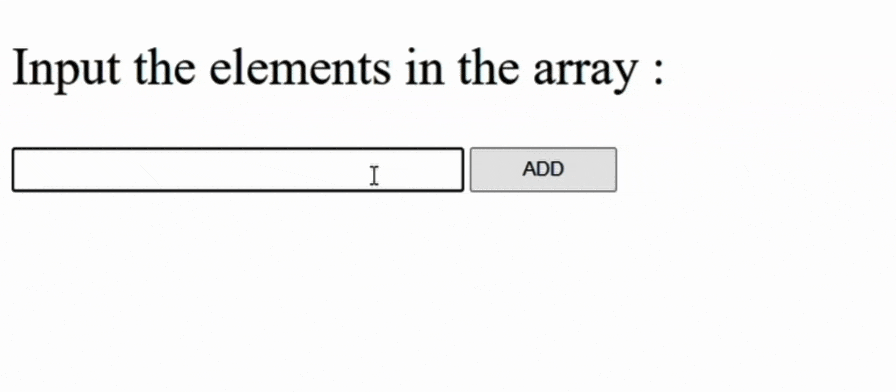# How to get index at which the value should be inserted into sorted array based on iterator function in JavaScript ?

In this article, we will learn how to get an index at which the value should be inserted into a sorted array based on the iterator function in Javascript. Here we use the sorting function to sort the array and we would print the array and the position of the new element in the DOM.

Approach:

• Create an empty array.
• Now make a sorting function by taking the parameter as an array.
• Now make an onClick function as whenever the button is pressed the function automatically runs.
• In the onClick function, we would first add the element to the array and then find the position of the element by using the iterator function
• In finding the position we would see if the array element is greater than or not if yes then mark that position and break the loop.
• Now at the end, we would print the array and the position of the newly added element into the DOM.

Example: In this example, we will use the above approach.

## HTML

 `` `<``html` `lang``=``"en"``>` `<``head``>` `    ``<``script` `src``=``"gfg.js"``>` `    ``<``style``>` `        ``input {` `            ``width: 300px;` `            ``height: 25px;` `        ``}` `        ``button {` `            ``width: 100px;` `            ``height: 31px;` `        ``}` `    ``` ``   `<``body``>` `    ``<``p` `style``=``"font-size: 35px;"``>` `        ``Input the elements in the array : ` `    ``` `    ``<``input` `type``=``"text"` `id``=``"element"``>` `    ``<``button` `type``=``"button"` `onclick``=``"add()"``>ADD` `    ``<``br``>` `    ``<``span` `id``=``"array"` `style``=``"font-size: 25px;"``>` `    ``<``br``>` `    ``<``span` `id``=``"new-position-array"` `          ``style``=``"font-size: 25px;"``>` `    ``` `` ``

## Javascript

 `//filename:gfg.js` `// Creating an empty array and when we add the` `// elements we increase the number of elements` `// by 1` `const arr = Array();` `let numberOfElements = 0;`   `// Sorting function to sort the array` `// Here we use the selection sort` `// algorithm for sorting` `function` `sort(arr) {` `    ``for` `(let i = 0; i < arr.length; i++) {` `        ``let min = i;` `        ``for` `(let j = i + 1; j < arr.length; j++) {` `            ``if` `(arr[j] < arr[min])` `                ``min = j;` `        ``}` `        ``let temp = arr[min];` `        ``arr[min] = arr[i];` `        ``arr[i] = temp;` `    ``}` `}` `// onClick function add` `function` `add() {`   `    ``// Taking the input from the text box to` `    ``// add the element to the given array` `    ``arr[numberOfElements] = parseInt(` `        ``document.getElementById(``"element"``).value);`   `    ``// Increasing the array length to add` `    ``// next number to the array` `    ``numberOfElements++;`   `    ``// Variables to find the position of` `    ``// the newly added element` `    ``let position = 0;` `    ``let flag = ``false``;`   `    ``// Finding the position of the newly added` `    ``// element in the sorted array` `    ``let newElement = arr[numberOfElements - 1];` `    ``for` `(let i = 0; i < arr.length; i++) {`   `        ``// If the array element is greater then` `        ``// the newly added element then mark that` `        ``// position and break the loop as this is` `        ``// the position of newly added element in` `        ``// the given sorted array` `        ``if` `(arr[i] > newElement) {` `            ``position = i;` `            ``flag = ``true``;` `            ``break``;` `        ``}` `    ``}` `    ``// If the newly added element is highest among` `    ``// the elements in the array then its position` `    ``// would be the array length - 1` `    ``if` `(flag == ``false``)` `        ``position = arr.length - 1;`   `    ``// Now calling the sort function to sort the` `    ``// given array after insertion` `    ``sort(arr);`   `    ``// For printing into the DOM` `    ``document.getElementById(``"element"``).value = ``""``;`   `    ``let print = ``"Array is : "``;`   `    ``for` `(let i = 0; i < arr.length; i++) {` `        ``print += arr[i] + ``" "``;` `    ``}` `    ``document.getElementById(``"array"``).innerHTML = print;` `    ``document.getElementById(``"new-position-array"``).innerHTML` `        ``= ``"New element: "` `+ newElement + ``" at Position: "` `        ``+ position;` `}`

Used Functions:

sort() Function: This function takes an argument of the desired array to be sorted and implements the selection sort and gives us the sorted array as a result.

add() Function: This function is an on-click function and it is triggered when the button is clicked. This function takes the value from the input box and stores it into the sorted array and increments the array index value by one. Then this function finds the position of the newly added element in the sorted array by comparing it with every element of the array and if it finds the value greater than it then it breaks the loop and stores the index of that position and then it calls the sort() function to sort the array again. Finally, it gives output in the DOM

Output:GIF of implementation

Whether you're preparing for your first job interview or aiming to upskill in this ever-evolving tech landscape, GeeksforGeeks Courses are your key to success. We provide top-quality content at affordable prices, all geared towards accelerating your growth in a time-bound manner. Join the millions we've already empowered, and we're here to do the same for you. Don't miss out - check it out now!

Previous
Next